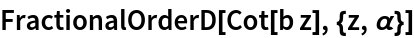#Function Repository Resource:

# FractionalOrderD

Compute the fractional derivative of an expression

Contributed by: Oleg Marichev & Paco Jain (Wolfram Research)
 ResourceFunction["FractionalOrderD"][f,{x,α}] gives the α-order fractional integro-derivative of f with respect to x.

## Details and Options

ResourceFunction["FractionalOrderD"] automatically maps over lists in its first argument.
All expressions that do not explicitly depend on the given variable are taken to have zero partial derivative.

## Examples

### Basic Examples (5)

Compute a fractional derivative of a power function:

 In:=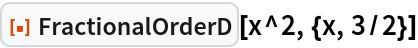Out=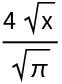The first-order fractional derivative is equivalent to differentiation:

 In:=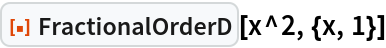Out=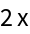In:=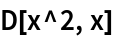Out=The negative-first-order fractional derivative is equivalent to indefinite integration:

 In:=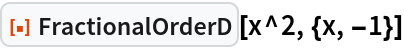Out=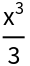In:=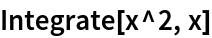Out=Compute the fractional derivative of symbolic order α of an exponential function:

 In:=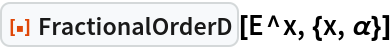Out=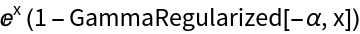Compute the fractional derivative of symbolic order α of a logarithmic function:

 In:=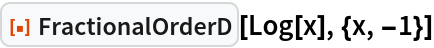Out=### Scope (1)

FractionalOrderD works with symbolic orders α:

 In:=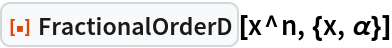Out=### Possible Issues (1)

FractionalOrderD may return results in terms of Unevaluated sums:

 In:=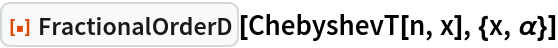Out=### Interactive Examples (1)

Varying α from 0 to -1 smoothly relates a function and its derivative:

 In:=Out=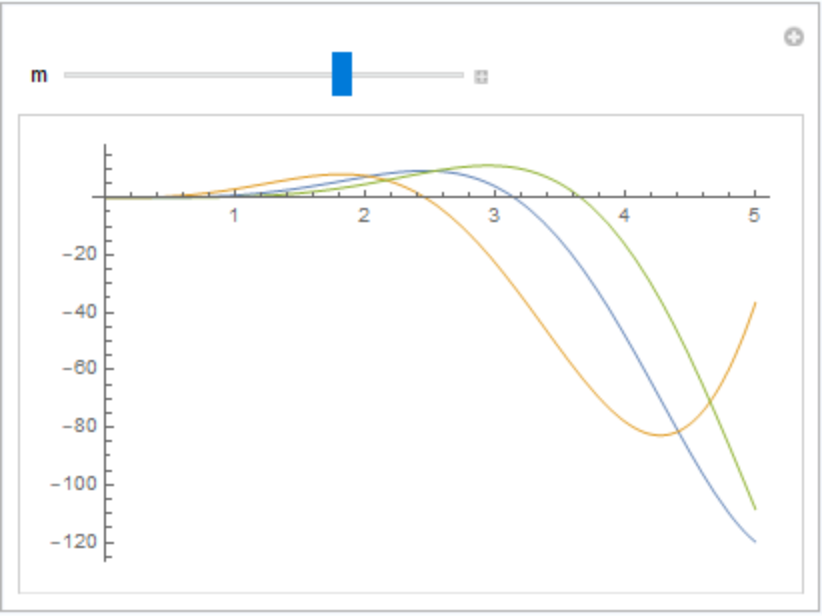### Neat Examples (1)

Create a table of fractional derivatives: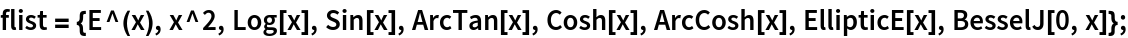In:=Out=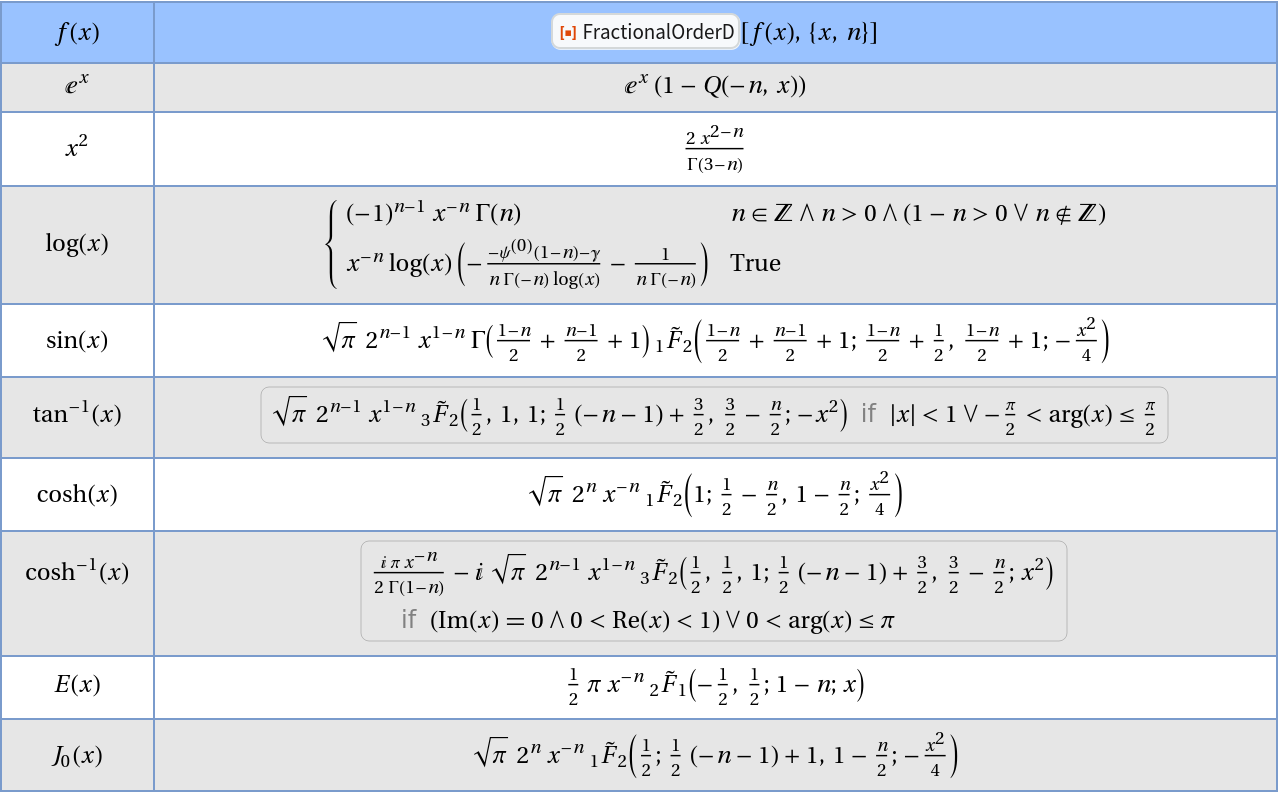## Author Notes

There are different ways of defining the fractional integro-derivative. The current implementation (v 1.0) implements only the Riemann-Liouville formulation. Another frequently used definition, the Grunwald-Letnikov formulation should perhaps be implemented in a future version.

### Known issues:

#### JSS Examples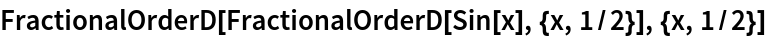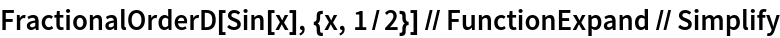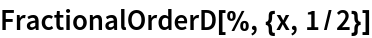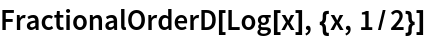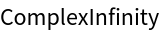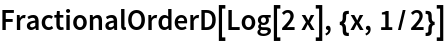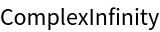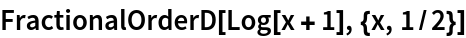#### Piecewising